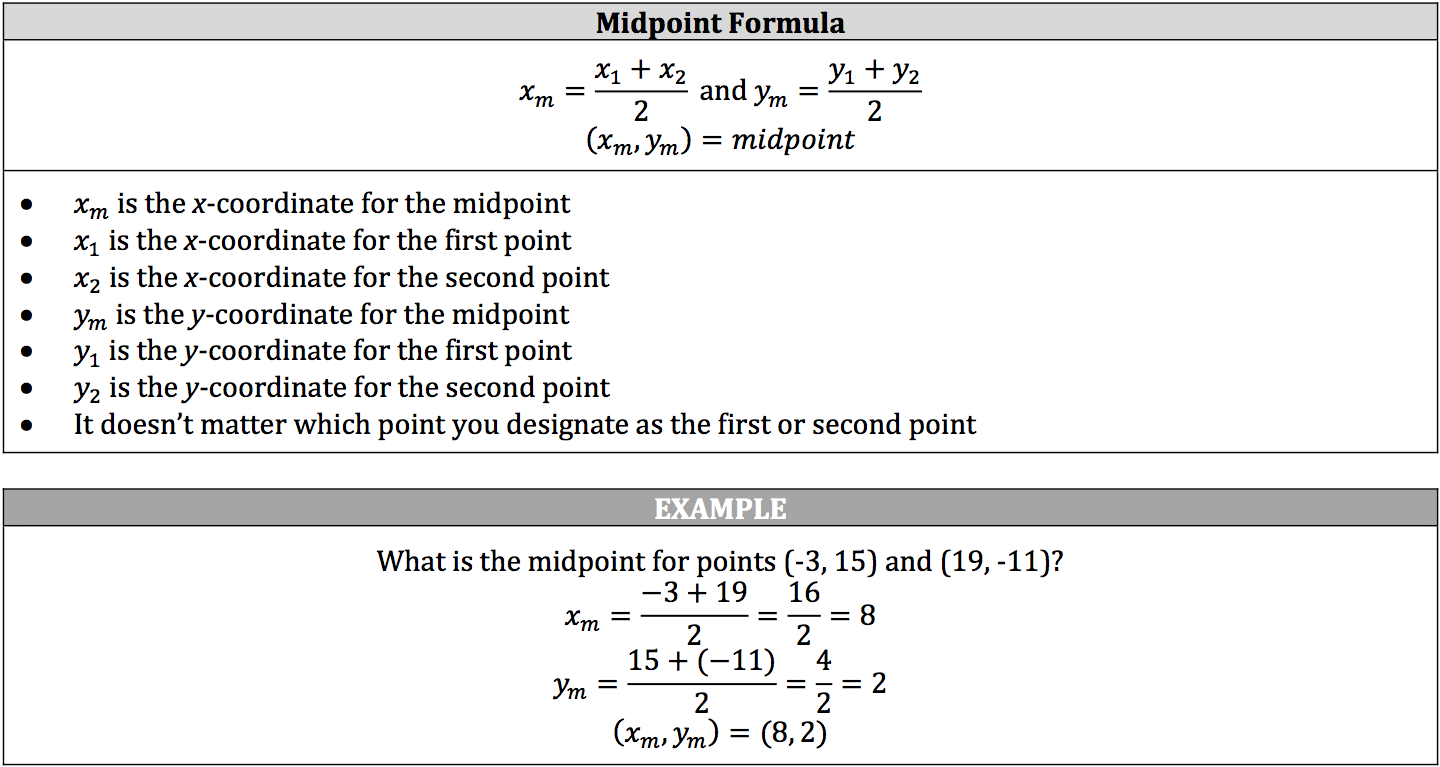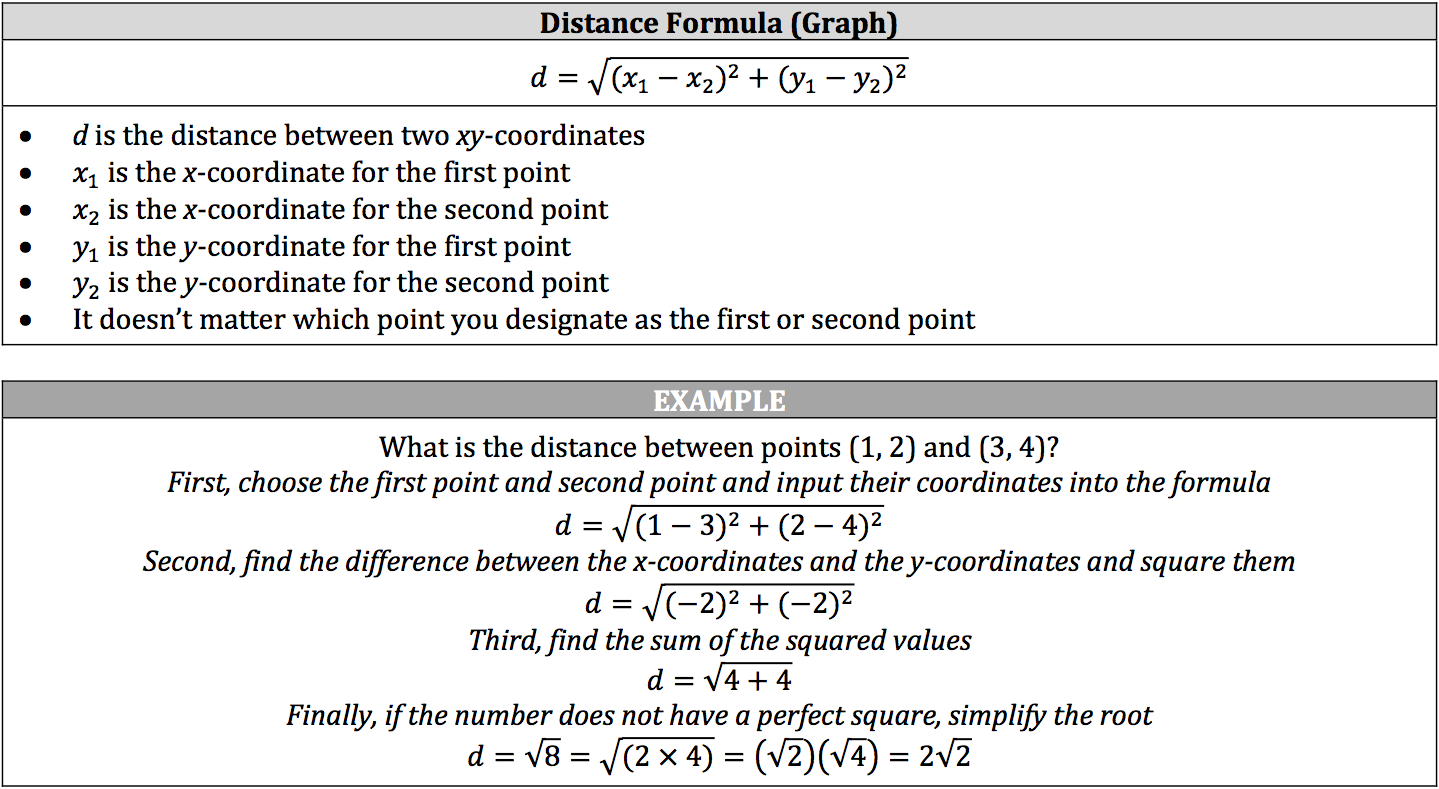The line graphs generated by linear equations are not limited to the portion of the graph shown, but instead continue on infinitely in either direction. Essentially, you are working with a segment of the line graph and a few of the points it contains. We can further analyze the measurements of these line segments through the midpoint formula and the distance formula:

• The midpoint formula measures the midpoint between two xy‐ coordinates.
• The distance formula for graphs measures the distance between two xy‐coordinates.

Note, do not confuse the distance formula with the formula for distance in distance, rate, and time measurements.

### Using the Midpoint Formula### Using the Distance Formula